## Sample Test Problems

1. A nucleus has a radius of 4 Fermis. Use the uncertainty principle to estimate the kinetic energy for a neutron localized inside the nucleus. Do the same for an electron.Try non-relativistic formula first and verify approximation when we have the energy.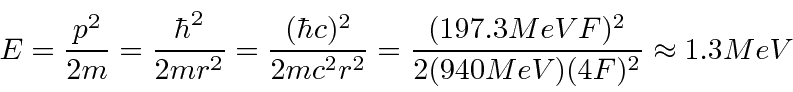This is much less than 940 MeV so the non-relativistic approximation is very good.

The electron energy will be higher and its rest mass is only 0.51 MeV so it WILL be relativistic. This makes it easier.2. * Assume that the potential for a neutron near a heavy nucleus is given byforFermis andforFermis. Use the uncertainty principle to estimate the minimum value ofneeded for the neutron to be bound to the nucleus.
3. Use the uncertainty principle to estimate the ground state energy of Hydrogen.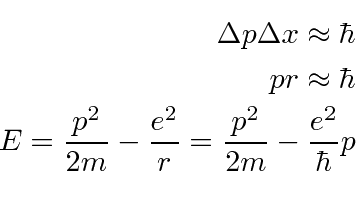(We could have replacedequally well.) Minimize.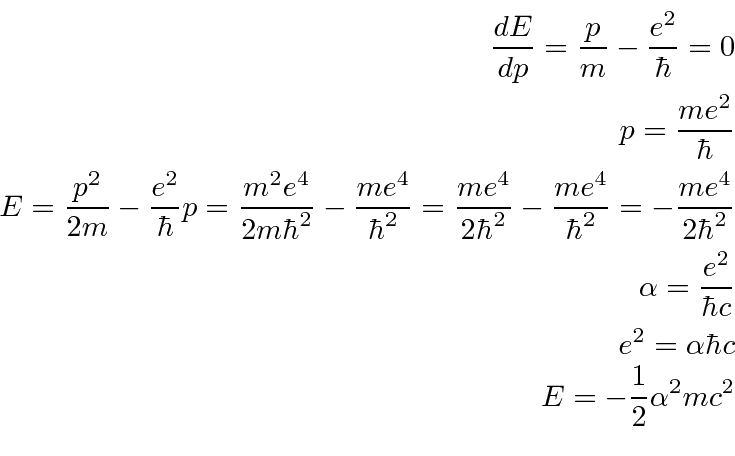4. * Given the following one dimensional probability amplitudes in the position variable x, compute the probability distribution in momentum space. Show that the uncertainty principle is roughly satisfied.
afor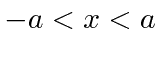, otherwise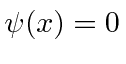.
b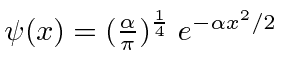c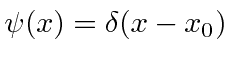5. Use the Heisenberg uncertainty principle to estimate the ground state energy for a particle of massin the potential.
6. * Find the one dimensional wave function in position spacethat corresponds to.
7. * Find the one dimensional wave function in position spacethat corresponds tofor, and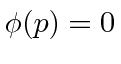otherwise.
8. * Assume that a particle is localized such thatfor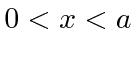and thatelsewhere. What is the probability for the particle to have a momentum betweenand?
9. A beam of photons of momentumis incident upon a slit of width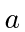. The resulting diffraction pattern is viewed on screen which is a distance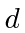from the slit. Use the uncertainty principle to estimate the width of the central maximum of the diffraction pattern in terms of the variables given.
10. * The wave-function of a particle in position space is given by. Find the wave-function in momentum space. Is the state correctly normalized? Explain why.
11. * A particle is in the state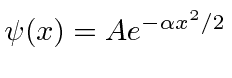. What is the probability for the particle to have a momentum betweenand?
12. A hydrogen atom has the potential. Use the uncertainty principle to estimate the ground state energy.
13. * Assume thatfor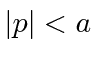andelsewhere. What is? What is the probability to find the particle betweenand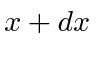?
14. The hydrogen atom is made up of a proton and an electron bound together by the Coulomb potential,. It is also possible to make a hydrogen-like atom from a proton and a muon. The force binding the muon to the proton is identical to that for the electron but the muon's mass is 106 MeV/c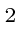. Use the uncertainty principle to estimate the energy and the radius of the ground state of muonic hydrogen.
15. * Given the following one dimensional probability amplitudes in the momentum representation, compute the probability amplitude in the position representation,. Show that the uncertainty principle is satisfied.
(a)for,elsewhere.
(b)(c)16. * Assume that. What is? What is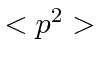? What is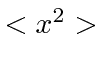?

Jim Branson 2013-04-22